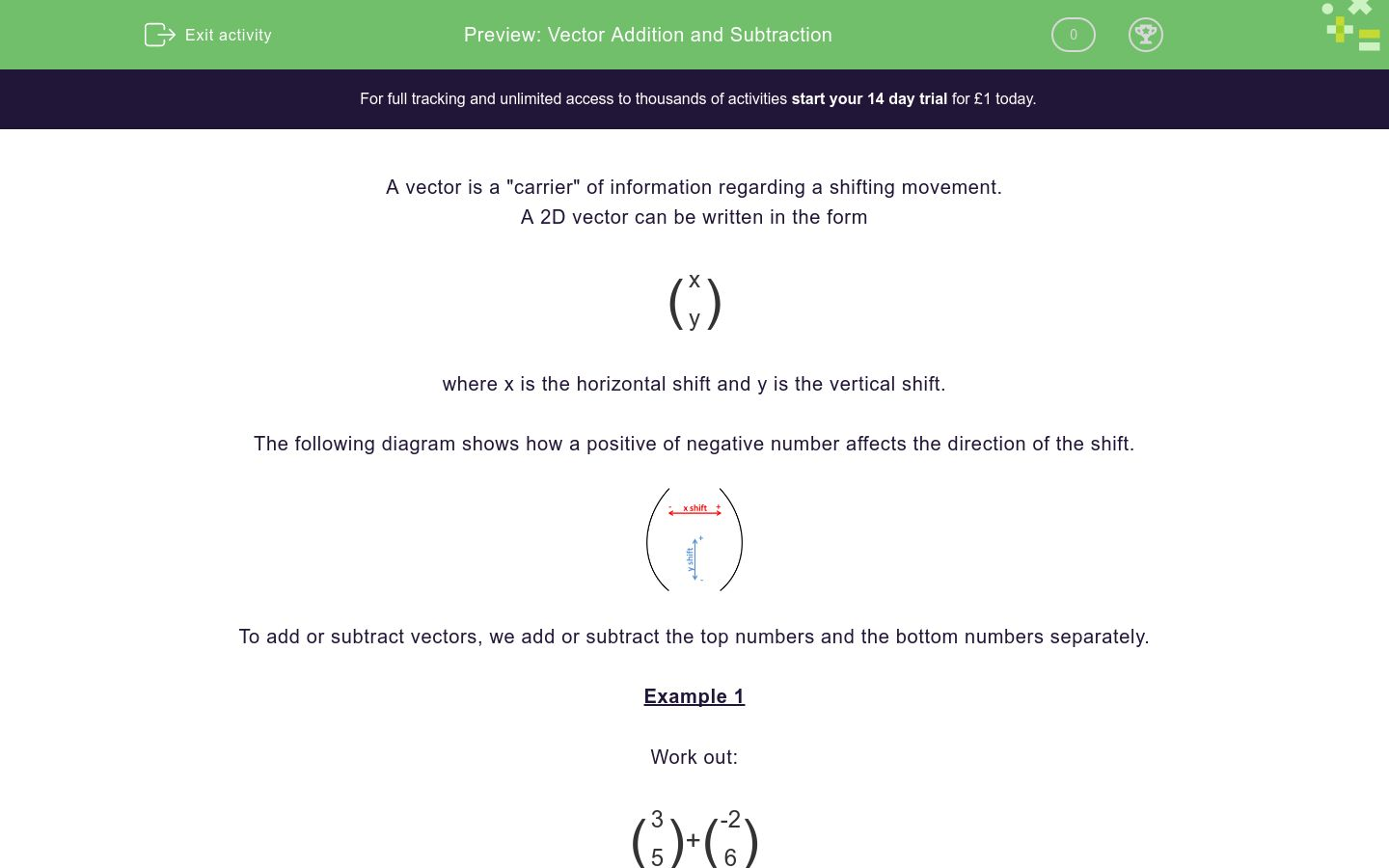# Vector Addition and Subtraction

In this worksheet, students add and subtract simple 2D vectors.Key stage:  KS 4

Curriculum topic:  Geometry and Measures

Difficulty level:### QUESTION 1 of 10

A vector is a "carrier" of information regarding a shifting movement.

A 2D vector can be written in the form

 ( x ) y

where x is the horizontal shift and y is the vertical shift.

The following diagram shows how a positive of negative number affects the direction of the shift.To add or subtract vectors, we add or subtract the top numbers and the bottom numbers separately.

Example 1

Work out:

 ( 3 ) + ( -2 ) 5 6

Answer

 ( 3 + -2 ) 5 + 6

 ( 1 ) 11

Example 2

Work out:

 ( 3 ) - ( -2 ) -5 6

Answer

 ( 3 - -2 ) -5 - 6

 ( 5 ) -11

Select the correct answer to:

 ( 4 ) + ( -2 ) 5 5

Answers

 1 ( 6 ) 2. ( 4 ) 3. ( 2 ) 10 10 10

1.

2.

3.

Select the correct answer to:

 ( 4 ) + ( -2 ) -5 5

Answers

 1 ( 2 ) 2. ( 4 ) 3. ( 2 ) 0 10 10

1.

2.

3.

Select the correct answer to:

 ( 5 ) - ( 2 ) 4 5

Answers

 1 ( 3 ) 2. ( 7 ) 3. ( 3 ) 1 9 -1

1.

2.

3.

Select the correct answer to:

 ( 5 ) - ( 1 ) 4 -5

Answers

 1 ( 4 ) 2. ( 4 ) 3. ( 3 ) 1 9 -1

1.

2.

3.

Select the correct answer to:

 ( 5 ) + ( 1 ) 4 -5

Answers

 1 ( 6 ) 2. ( 4 ) 3. ( 6 ) 1 9 -1

1.

2.

3.

Select the correct answer to:

 ( -5 ) + ( -1 ) 4 -5

Answers

 1 ( -6 ) 2. ( -6 ) 3. ( 6 ) 1 -1 -1

1.

2.

3.

Select the correct answer to:

 ( -5 ) - ( -1 ) 4 -5

Answers

 1 ( -6 ) 2. ( -6 ) 3. ( -4 ) 1 -1 9

1.

2.

3.

Select the correct answer to:

 ( -3 ) - ( -3 ) 3 0

Answers

 1 ( 0 ) 2. ( -6 ) 3. ( 6 ) 3 3 3

1.

2.

3.

Select the correct answer to:

 ( -8 ) + ( -3 ) 3 -5

Answers

 1 ( 11 ) 2. ( -11 ) 3. ( -5 ) 2 -2 2

1.

2.

3.

Select the correct answer to:

 ( -8 ) - ( -3 ) -3 -5

Answers

 1 ( 11 ) 2. ( -11 ) 3. ( -5 ) 2 -2 2

1.

2.

3.

• Question 1

Select the correct answer to:

 ( 4 ) + ( -2 ) 5 5

Answers

 1 ( 6 ) 2. ( 4 ) 3. ( 2 ) 10 10 10

CORRECT ANSWER
3.
• Question 2

Select the correct answer to:

 ( 4 ) + ( -2 ) -5 5

Answers

 1 ( 2 ) 2. ( 4 ) 3. ( 2 ) 0 10 10

CORRECT ANSWER
1.
• Question 3

Select the correct answer to:

 ( 5 ) - ( 2 ) 4 5

Answers

 1 ( 3 ) 2. ( 7 ) 3. ( 3 ) 1 9 -1

CORRECT ANSWER
3.
• Question 4

Select the correct answer to:

 ( 5 ) - ( 1 ) 4 -5

Answers

 1 ( 4 ) 2. ( 4 ) 3. ( 3 ) 1 9 -1

CORRECT ANSWER
2.
• Question 5

Select the correct answer to:

 ( 5 ) + ( 1 ) 4 -5

Answers

 1 ( 6 ) 2. ( 4 ) 3. ( 6 ) 1 9 -1

CORRECT ANSWER
3.
• Question 6

Select the correct answer to:

 ( -5 ) + ( -1 ) 4 -5

Answers

 1 ( -6 ) 2. ( -6 ) 3. ( 6 ) 1 -1 -1

CORRECT ANSWER
2.
• Question 7

Select the correct answer to:

 ( -5 ) - ( -1 ) 4 -5

Answers

 1 ( -6 ) 2. ( -6 ) 3. ( -4 ) 1 -1 9

CORRECT ANSWER
3.
• Question 8

Select the correct answer to:

 ( -3 ) - ( -3 ) 3 0

Answers

 1 ( 0 ) 2. ( -6 ) 3. ( 6 ) 3 3 3

CORRECT ANSWER
1.
• Question 9

Select the correct answer to:

 ( -8 ) + ( -3 ) 3 -5

Answers

 1 ( 11 ) 2. ( -11 ) 3. ( -5 ) 2 -2 2

CORRECT ANSWER
2.
• Question 10

Select the correct answer to:

 ( -8 ) - ( -3 ) -3 -5

Answers

 1 ( 11 ) 2. ( -11 ) 3. ( -5 ) 2 -2 2

CORRECT ANSWER
3.
---- OR ----

Sign up for a £1 trial so you can track and measure your child's progress on this activity.

### What is EdPlace?

We're your National Curriculum aligned online education content provider helping each child succeed in English, maths and science from year 1 to GCSE. With an EdPlace account you’ll be able to track and measure progress, helping each child achieve their best. We build confidence and attainment by personalising each child’s learning at a level that suits them.

Start your £1 trial